Tag: groups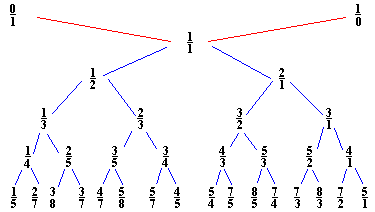John Farey (1766-1826) was a geologist of sorts. Eyles, quoted on the math-biographies site described his geological work as

“As a geologist Farey is entitled to respect for the work which he carried out himself, although it has scarcely been noticed in the standard histories of geology.”

That we still remember his name after 200 years is due to a short letter he wrote in 1816 to the editor of the Philosophical Magazine

“On a curious Property of vulgar Fractions.
By Mr. J. Farey, Sen. To Mr. Tilloch

Sir. – On examining lately, some very curious and elaborate Tables of “Complete decimal Quotients,” calculated by Henry Goodwyn, Esq. of Blackheath, of which he has printed a copious specimen, for private circulation among curious and practical calculators, preparatory to the printing of the whole of these useful Tables, if sufficient encouragement, either public or individual, should appear to warrant such a step: I was fortunate while so doing, to deduce from them the following general property; viz.

If all the possible vulgar fractions of different values, whose greatest denominator (when in their lowest terms) does not exceed any given number, be arranged in the order of their values, or quotients; then if both the numerator and the denominator of any fraction therein, be added to the numerator and the denominator, respectively, of the fraction next but one to it (on either side), the sums will give the fraction next to it; although, perhaps, not in its lowest terms.

For example, if 5 be the greatest denominator given; then are all the possible fractions, when arranged, 1/5, 1/4, 1/3, 2/5, 1/2, 3/5, 2/3, 3/4, and 4/5; taking 1/3, as the given fraction, we have (1+1)/(5+3) = 2/8 = 1/4 the next smaller fraction than 1/3; or (1+1)/(3+2) = 2/5, the next larger fraction to 1/3. Again, if 99 be the largest denominator, then, in a part of the arranged Table, we should have 15/52, 28/97, 13/45, 24/83, 11/38, &c.; and if the third of these fractions be given, we have (15+13)/(52+45) = 28/97 the second: or (13+11)/(45+38) = 24/83 the fourth of them: and so in all the other cases.

I am not acquainted, whether this curious property of vulgar fractions has been before pointed out?; or whether it may admit of any easy or general demonstration?; which are points on which I should be glad to learn the sentiments of some of your mathematical readers; and am

J. Farey. Howland-street.”

So, if we interpolate “childish addition of fractions” $\frac{a}{b} \oplus \frac{c}{d} = \frac{a+c}{b+d}$ and start with the numbers $0 = \frac{0}{1}$ and $\infty = \frac{1}{0}$ we get the binary Farey-tree above. For a fixed natural number n, if we stop the interpolation whenever the denominator of the fraction would become larger than n and order the obtained fractions (smaller or equal to one) we get the Farey sequence F(n). For example, if n=3 we start with the sequence $\frac{0}{1},\frac{1}{1}$. The next step we get $\frac{0}{1},\frac{1}{2},\frac{1}{1}$ and the next step gives

$\frac{0}{1},\frac{1}{3},\frac{1}{2},\frac{2}{3},\frac{1}{1}$

and as all the denomnators of childish addition on two consecutive fractions will be larger than 3, the above sequence is F(3). A remarkable feature of the series F(n) is that if $\frac{a}{b}$ and $\frac{c}{d}$ are consecutive terms in F(n), then

$det \begin{bmatrix} a & c \\\ b & d \end{bmatrix} = -1$

and so these two fractions are the endpoints of an even geodesic in the Dedekind tessellation.

A generalized Farey series is an ordered collection of fractions $\infty,x_0,x_1,\cdots,x_n,\infty$ such that $x_0$ and $x_n$ are integers and some $x_i=0$. Moreover, writing $x_i = \frac{a_i}{b_i}$ we have that

$det \begin{bmatrix} a_i & a_{i+1} \\\ b_i & b_{i+1} \end{bmatrix} = -1$

A Farey code is a generalized Farey sequence consisting of all the vertices of a special polygon that lie in $\mathbb{R} \cup \{ \infty \}$ together with side-pairing information. If two consecutive terms are such that the complete geodesic between $x_i$ and $x_{i+1}$ consists of two sides of the polygon which are paired we denote this fact by
$$\xymatrix{x_i \ar@{-}[r]_{\circ} & x_{i+1}}$$. If they are the endpoints of two odd sides of the polygon which are paired we denote this by $$\xymatrix{x_i \ar@{-}[r]_{\bullet} & x_{i+1}}$$. Finally, if they are the endpoints of a free side which is paired to another free side determined by $x_j$ and $x_{j+1}$ we denote this fact by marking both edges $$\xymatrix{x_i \ar@{-}[r]_{k} & x_{i+1}}$$ and $$\xymatrix{x_j \ar@{-}[r]_{k} & x_{j+1}}$$ with the same number.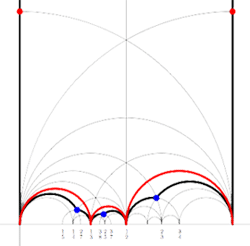For example, for the M(12) special polygon on the left (bounded by the thick black geodesics), the only vertices in $\mathbb{R} \cup \{ \infty \}$ are $\infty,0,\frac{1}{3},\frac{1}{2},1$. The two vertical lines are free sides and are paired, whereas all other sides of the polygon are odd. Therefore the Farey-code for this Mathieu polygon is

$$\xymatrix{\infty \ar@{-}[r]_{1} & 0 \ar@{-}[r]_{\bullet} & \frac{1}{3} \ar@{-}[r]_{\bullet} & \frac{1}{2} \ar@{-}[r]_{\bullet} & 1 \ar@{-}[r]_{1} & \infty}$$

Conversely, to a Farey-code we can associate a special polygon by first taking the hyperbolic convex hull of all the terms in the sequence (the region bounded by the vertical lines and the bottom red circles in the picture on the left) and adding to it for each odd interval $$\xymatrix{x_i \ar@{-}[r]_{\bullet} & x_{i+1}}$$ the triangle just outside the convex hull consisting of two odd edges in the Dedekind tessellation (then we obtain the region bounded by the black geodesics). Again, the side-pairing of the obained special polygon can be obtained from that of the Farey-code.

This correspondence gives a natural one-to-one correspondence special polygons < ---> Farey-codes . _Later_ we will see how the Farey-code determines the group structure of the corresponding finite index subgroup of the modular group $\Gamma = PSL_2(\mathbb{Z})$.

Reference

Ravi S. Kulkarni, “An arithmetic-geometric method in the study of the subgroups of the modular group”, Amer. J. Math 113 (1991) 1053-1133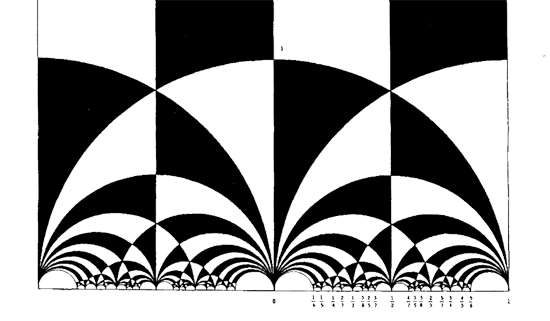In 1877, Richard Dedekind discovered one of the most famous pictures in mathematics : the black&white tessellation of the upper half-plane in hyperbolic triangles. Recall that the group $SL_2(\mathbb{Z})$ of all invertible 2×2 integer matrices with determinant $1$ acts on the upper halfplane via

From time to time you may see here a message that NeverEndingBooks ends on Bloomsday (June 16th). Soon after, I hope to restart with another blog at the same URL. For starters, Neverendingbooks refers to my never-ending bookproject on noncommutative geometry started in 1999, a millenium ago… Today I\’m correcting the proofs and have even seen the cover-design of the book, supposed to be published in the fall. So, it should be really EndingBook(s), finally. From time to time it is good to start afresh. The next project is still pretty vague to me but it will be a lot more focussed and center around topics like Moonshine, the Monster, the Mathieu groups, Modular forms and group etc. Suggestions for a blogtitle are welcome (M-theory is already taken…). Besides there are technical problems with the machine running the blog, a new one is expected around June 16th. As I will not be able to clone between the two (one PPC, the new one Intel) I decided to start again from scratch. Anyway, Ive made a database-dump of NeverEndingBooks and will make it available to anyone interested in reading old posts (even the ones with a private-status). Finally, there are other reasons, better kept private. Give me a couple of weeks to resurface. For now, all the best.

Someone from down under commented on the group think post yesterday :

Nice post, but I might humbly suggest that there’s not much in it that anyone would disagree with. I’d be interested in your thoughts on the following:
1. While many doomed research programs have the seven symptoms you mention, so do some very promising research programs. For instance, you could argue that Grothendieck’s school did. While it did eventually explode, it remains one of the high points in the history of mathematics. But at the time, many people (Mordell, Siegel) thought it was all garbage. Indeed there was even doubt into the late eighties. Is there anything close to a necessary and sufficient condition that an outsider can use to get some idea of whether a research group is doing work that will last?
2. Pretty much everyone thinks they’re underappreciated. It’s easy to advise them to pull a Perelman because it costs you nothing. But most unappreciated researchers are unappreciated for a good reason. How can unappreciated researchers decide whether their ideas really are good or not before spending ten years of their lives finding out?

First the easy bit : the ‘do a Perelman’-sentence seems to have been misread by several people (probably due to my inadequate English). I never suggested ‘unappreciated researchers’ to pull a Perelman but rather the key figures in seemingly successful groups making outrageous claims for power-reasons. Here is what I actually wrote

An aspect of these groupthinking science-groups that worries me most of all is their making of exagerated claims to potential applications, not supported (yet) by solid proof. Short-time effect may be to attract more people to the subject and to keep doubting followers on board, but in the long term (at least if the claimed results remain out of reach) this will destroy the subject itself (and, sadly enough, also closeby subjects making no outrageous claims!). My advice to people making such claims is : do a Perelman! Rather than doing a PR-job, devote yourself for as long as it takes to prove your hopes, somewhere in splendid isolation and come back victoriously. I have a spare set of keys if you are in search for the perfect location!
Before I will try to answer both questions let me stress that this is just my personal opinion to which I attach no particular value. Sure, I will forget things and will over-stress others. You can always leave a comment if you think I did, but I will not enter a discussion. I think it is important that a person develops his or her own scientific ethic and tries to live by it. 1. Is there anything close to a necessary and sufficient condition that an outsider can use to get some idea of whether a research group is doing work that will last? Clearly, the short answer to this is “no”. Still, there are some signs an outsider might pick up to form an opinion. – What is the average age of the leading people in the group? (the lower, the better) – The percentage of talks given by young people at a typical conference of the group (the higher, the better) – The part of a typical talk in the subject spend setting up notation, referring to previous results and namedropping (the lower, the better) – The number of group-outsiders invited to speak at a typical conference (the higher, the better) – The number of self-references in a typical paper (the lower, the better) – The number of publications by the group in non-group controlled journals (the higher, the better) – The number of group-controlled journals (the lower, the better) – The readablity of survey papers and textbooks on the subject (the higher, the better) – The complexity of motivating examples not covered by competing theories (the lower, the better) – The number of subject-gurus (the higher, the better) – The number of phd-students per guru (the lower, the better) – The number of main open problems (the higher, the better) – The Erdoes-like number of a typical group-member wrt. John Conway (the lower, the better) Okay, Im starting to drift but I hope you get the point. It is not that difficult to set up your own tools to measure the amount to which a scientific group suffers from group think. Whether the group will make a long-lasting contribution is another matter which is much harder to predict. Here, I would go for questions like : – Does the theory offer a new insight into classical & central mathematical objects such as groups, curves, modular forms, Dynkin diagrams etc. ? – Does the theory offer tools to reduce the complexity of a problem or does is instead add a layer of technical complexity? That is, are they practicing mathematics or obscurification? 2. How can unappreciated researchers decide whether their ideas really are good or not before spending ten years of their lives finding out? Here is my twofold advice to all the ‘unappreciated’ : (1) be at least as critical to your own work as you are to that of others (it is likely you will find out that you are rightfully under-appreciated compared to others) and (2) enjoy the tiny tokens of appreciation because they are likely all that you will ever get. Speaking for myself, I do not feel unappreciated compared to what I did. I did prove a couple of good results to which adequate reference is given and I had a couple of crazy ideas which were ridiculed by some at the time. A silly sense of satisfaction comes from watching the very same people years later fall over each other trying to reclaim some of the credit for these ideas. Okay, it may not have the same status of recognition as a Fields medal or a plenary talk at the ICM but it is enough to put a smile on my face from time to time and to continue stubbornly with my own ideas.

After a lengthy spring-break, let us continue with our course on noncommutative geometry and $SL_2(\mathbb{Z})$-representations. Last time, we have explained Grothendiecks mantra that all algebraic curves defined over number fields are contained in the profinite compactification
$\widehat{SL_2(\mathbb{Z})} = \underset{\leftarrow}{lim}~SL_2(\mathbb{Z})/N$ of the modular group $SL_2(\mathbb{Z})$ and in the knowledge of a certain subgroup G of its group of outer automorphisms
. In particular we have seen that many curves defined over the algebraic numbers $\overline{\mathbb{Q}}$ correspond to permutation representations of $SL_2(\mathbb{Z})$. The profinite compactification $\widehat{SL_2}=\widehat{SL_2(\mathbb{Z})}$ is a continuous group, so it makes sense to consider its continuous n-dimensional representations $\mathbf{rep}_n^c~\widehat{SL_2}$ Such representations are known to have a finite image in $GL_n(\mathbb{C})$ and therefore we get an embedding $\mathbf{rep}_n^c~\widehat{SL_2} \hookrightarrow \mathbf{rep}_n^{ss}~SL_n(\mathbb{C})$ into all n-dimensional (semi-simple) representations of $SL_2(\mathbb{Z})$. We consider such semi-simple points as classical objects as they are determined by – curves defined over $\overline{Q}$ – representations of (sporadic) finite groups – modlart data of fusion rings in RCTF – etc… To get a feel for the distinction between these continuous representations of the cofinite completion and all representations, consider the case of $\hat{\mathbb{Z}} = \underset{\leftarrow}{lim}~\mathbb{Z}/n \mathbb{Z}$. Its one-dimensional continuous representations are determined by roots of unity, whereas all one-dimensional (necessarily simple) representations of $\mathbb{Z}=C_{\infty}$ are determined by all elements of $\mathbb{C}$. Hence, the image of $\mathbf{rep}_1^c~\hat{\mathbb{Z}} \hookrightarrow \mathbf{rep}_1~C_{\infty}$ is contained in the unit circle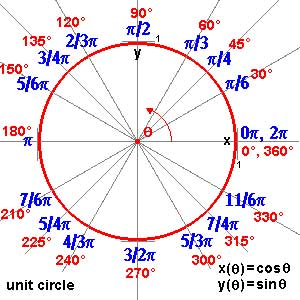and though these points are very special there are enough of them (technically, they form a Zariski dense subset of all representations). Our aim will be twofold : (1) when viewing a classical object as a representation of $SL_2(\mathbb{Z})$ we can define its modular content (which will be the noncommutative tangent space in this classical point to the noncommutative manifold of $SL_2(\mathbb{Z})$). In this way we will associate noncommutative gadgets to our classical object (such as orders in central simple algebras, infinite dimensional Lie algebras, noncommutative potentials etc. etc.) which give us new tools to study these objects. (2) conversely, as we control the tangentspaces in these special points, they will allow us to determine other $SL_2(\mathbb{Z})$-representations and as we vary over all classical objects, we hope to get ALL finite dimensional modular representations. I agree this may all sound rather vague, so let me give one example we will work out in full detail later on. Remember that one can reconstruct the sporadic simple Mathieu group $M_{24}$ from the dessin d’enfant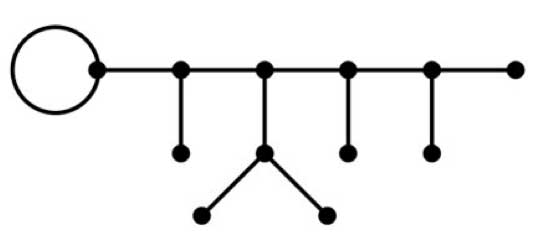This
dessin determines a 24-dimensional permutation representation (of
$M_{24}$ as well of $SL_2(\mathbb{Z})$) which
decomposes as the direct sum of the trivial representation and a simple
23-dimensional representation. We will see that the noncommutative
tangent space in a semi-simple representation of
$SL_2(\mathbb{Z})$ is determined by a quiver (that is, an
oriented graph) on as many vertices as there are non-isomorphic simple
components. In this special case we get the quiver on two points
$\xymatrix{\vtx{} \ar@/^2ex/[rr] & & \vtx{} \ar@/^2ex/[ll] \ar@{=>}@(ur,dr)^{96} }$ with just one arrow in each direction
between the vertices and 96 loops in the second vertex. To the
experienced tangent space-reader this picture (and in particular that
there is a unique cycle between the two vertices) tells the remarkable
fact that there is **a distinguished one-parameter family of
24-dimensional simple modular representations degenerating to the
permutation representation of the largest Mathieu-group**. Phrased
differently, there is a specific noncommutative modular Riemann surface
associated to $M_{24}$, which is a new object (at least as far
as I’m aware) associated to this most remarkable of sporadic groups.
Conversely, from the matrix-representation of the 24-dimensional
permutation representation of $M_{24}$ we obtain representants
of all of this one-parameter family of simple
$SL_2(\mathbb{Z})$-representations to which we can then perform
noncommutative flow-tricks to get a Zariski dense set of all
24-dimensional simples lying in the same component. (Btw. there are
also such noncommutative Riemann surfaces associated to the other
what is the noncommutative manifold of $SL_2(\mathbb{Z})$? and what is its connection with the Kontsevich-Soibelman coalgebra? (12)
is there a noncommutative compactification of $SL_2(\mathbb{Z})$? (and other arithmetical groups) (13) : how does one calculate the noncommutative curves associated to the Mathieu groups? (14) : whatever comes next… (if anything).